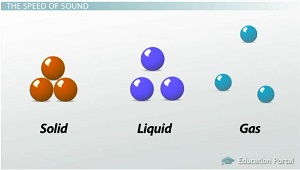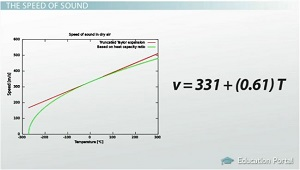# What is Sound? – Definition and Factors Affecting the Speed of Sound

Jan 9, 2020 | Sound

Explore the minute workings of sound waves and how they travel. We’ll discuss the main factors that affect the speed of sound waves, and we’ll try some mathematical problems involving the wave equation.

## The Nature of Sound Waves

Are you one of those people who likes to shout, ‘Echo!’ whenever you’re inside a cavernous building? I think it’s fun to experiment with echoes. Lots of people like to shout when they’re standing under a bridge, inside a parking garage, or at the bottom of a canyon.

We know that echoes are simply the reflection of sound from the rigid walls of buildings and natural formations. But what can echoes tell us about sound waves? Can we learn how sound travels from one place to another by studying the echo phenomenon?

Before we get into that, let’s review what a sound wave is. We’ve learned already that sound waves are longitudinal waves. That is, the particles in the medium vibrate in a direction parallel to the movement of the wave. Longitudinal waves are tougher to visualize than transverse waves. So let’s use the sound of a guitar string as an example.

## The Medium

When you pluck a guitar string, it begins to vibrate from side to side. This is what it would look like in slow motion. Let’s pretend these are the air particles that are evenly distributed around the guitar and everywhere else. When the string moves to the right, like this, it pushes on the air particles and makes them press together. This is called a compression.When the string moves to the left, like this, it creates a gap in the air particles and they get farther apart. This is called a rarefaction. You probably remember that compressions and rarefactions are the equivalent of crests and troughs for a longitudinal wave. These areas of higher and lower air pressure travel outward, away from the string. In other words, the sound wave travels outward from the guitar. However, the air particles themselves do not travel. They simply oscillate back and forth, in a direction parallel to the motion of the sound wave.

In this example, the air surrounding the guitar is called the medium, because it’s the material that carries the sound. When talking about waves, a medium is the substance that transports a wave from one place to another. The medium for sound waves is often air. But sound can also travel through liquids and solids.If you’re talking about sound waves traveling between two whales singing in the ocean, then the medium is seawater. If you’re talking about your neighbor’s music traveling through the walls of his house, then the medium is the wood and drywall. No matter what the medium is, sound waves are able to travel through it because of the particles interacting with each other. The compressions and rarefactions occur within the particles of seawater, wood, or drywall, just as they did in the air outside our guitar string.

Once the first particles are pushed by the vibrations of the sound source, then they pass this push on to the next particles, which pass it on to the next, and so on. Thus the wave travels through the medium as a result of the particles bumping back and forth on each other. The interactions between particles in the medium cause the sound wave to travel through the medium.

So, if sound waves travel because of the particles in the medium, then what happens to sound if there is no medium? Well, you guessed it. Without a medium, there’s no sound. Sound waves require a medium in which to travel from one place to another.

Think about a place where there’s absolutely no air, like the surface of the moon. If you were standing on the moon, and your friend was standing ten feet away from you, you could shout and scream and clap your hands, and your friend wouldn’t hear a thing. The moon has no atmosphere; it’s in the great vacuum of outer space. So it doesn’t matter how much noise you make – your sound will not carry anywhere, because there’s no medium to carry it.

## The Speed of Sound

Let’s go back to the different mediums that sound can travel through. We said that sound waves travel through solids, liquids, and gases. They can travel through all states of matter. But do the waves travel the same way through each of these different substances?We know that a solid has its particles packed very tightly together. Liquid particles are more loosely packed, and particles in a gas are pretty far apart. The distance between the particles affects how strong the interactions are between them. This, in turn, affects how quickly they transfer the energy of the wave. The stronger the particle interactions are, the more quickly the wave is transferred. So in general, sound travels faster in solids than in liquids, and faster in liquids than in gas.

Temperature actually increases the speed of sound, because warmer particles generally move at a faster rate. Scientists have derived a special formula for finding the speed of sound in dry air. It works for most of the temperatures found on Earth. The formula is v = 331 + (0.61)T, where v is the speed of sound, and T is the temperature.

For this formula, speed must be measured in meters per second, and temperature in degrees Celsius. You can see by this formula that an increase in temperature, or T, would cause an increase in speed, or v. If the temperature was 20° C, then the speed of sound in dry air would be about 343 meters per second. If the temperature rose to 30° C, then the speed would increase to 349 meters per second.## Speed and the Wave Equation

Don’t forget that the speed of sound has everything to do with what’s happening with the sound wave. A wave is a disturbance traveling through a medium. When we measure the speed of sound, what we’re really finding is how fast a disturbance is passed between particles, or the distance a disturbance travels per unit of time.

We just saw one formula for finding the speed of sound, but most formulas for sound speed are much more complicated. There are so many factors out there that can change the speed of sound, there’s no one formula that accounts for them all. But when it comes down to wave speed, all you’re really looking for is distance per unit time, right? So I wonder if we can find the speed of a sound wave based on the other wave parameters.

Do you remember this formula: v = lambda * f? It’s actually called the wave equation. It states that you can find the speed of a wave by multiplying the wavelength by the frequency. You can also rearrange this to find speed by dividing wavelength by the period because period is the reciprocal of frequency.

Let’s say the wavelength for a sound wave was 34 meters and it took exactly one tenth of a second to pass by. The wavelength would be 34, and the period would be 0.1. So the speed of this wave would be 340 meters per second.

Let’s try it using frequency. Let’s say the frequency of a sound wave is 500 Hertz; that is, 500 wave cycles per second, and the wavelength is 0.7 meters. To find the speed, you’d multiply these two and get 350 meters per second..So, do you think this wave equation can help us learn anything about echoes? If you have ever been inside a very steep-walled canyon, you know that sound can bounce around between the stone walls. If you shout, you’ll hear your own call return to you in a matter of seconds. If you could time exactly how many seconds your echo took, could you find the speed of sound inside the canyon? Let’s try it.

You’re standing in a canyon, facing a vertical wall 519 meters away. You let out a shout, and it returns to you in exactly 3 seconds. What’s the speed of sound in this case? Well, you know the time it took, so the period is 3 seconds. The distance the wave traveled was 519 – no, it was 1038 meters! You have to double the distance because the wave traveled to the canyon wall and returned back to you. So if speed is meters per second, then 1038 meters divided by 3 seconds is 346 meters per second, and that sounds like a reasonable speed for a sound wave.

## Lesson Summary

Sound waves are longitudinal waves that travel by compressions and rarefactions in the air, water, or other mediums. Sound requires a medium in order to travel; therefore it cannot travel in a vacuum. Sound waves travel faster through solids than liquids – and faster through liquids than gas. The speed of sound waves increases with higher temperatures but decreases with higher densities.

A simple way to find the speed of a sound wave is to use the wave equation, where speed is derived from wavelength and frequency or period. Sound speed can also be found by timing an echo from a known distance. While many factors affect how fast a sound wave travels, sound speed really comes down to how the particles interact inside of the medium.

## Learning Outcomes

After watching this lesson, you should be able to:

• Describe compressions and rarefactions
• Define medium and explain how the state of matter, density, and temperature of the mediums affect sound waves
• Recall the wave equation and use it to find the speed of sound